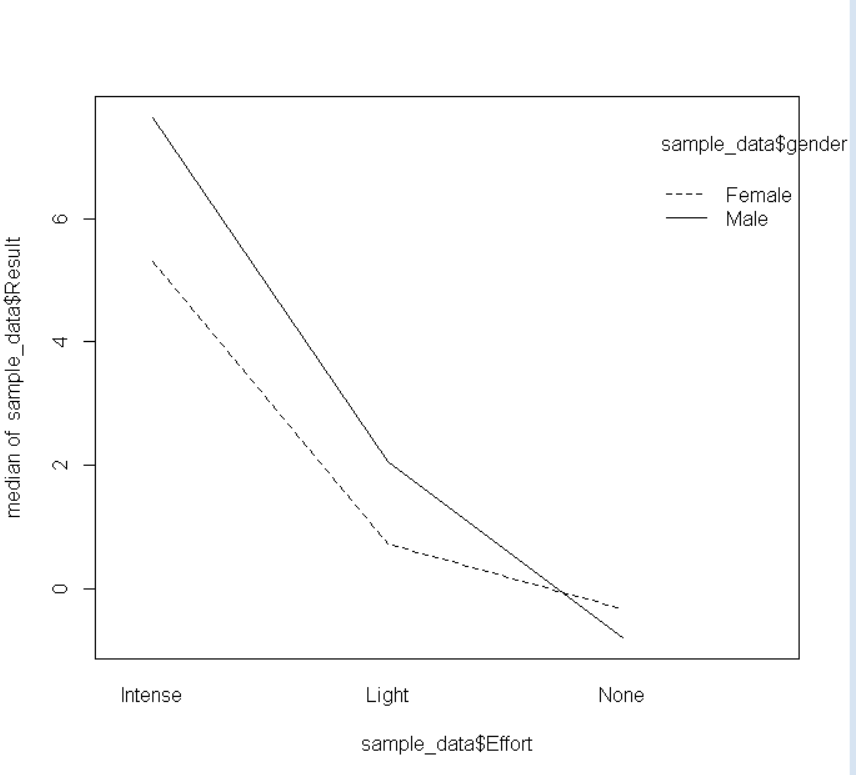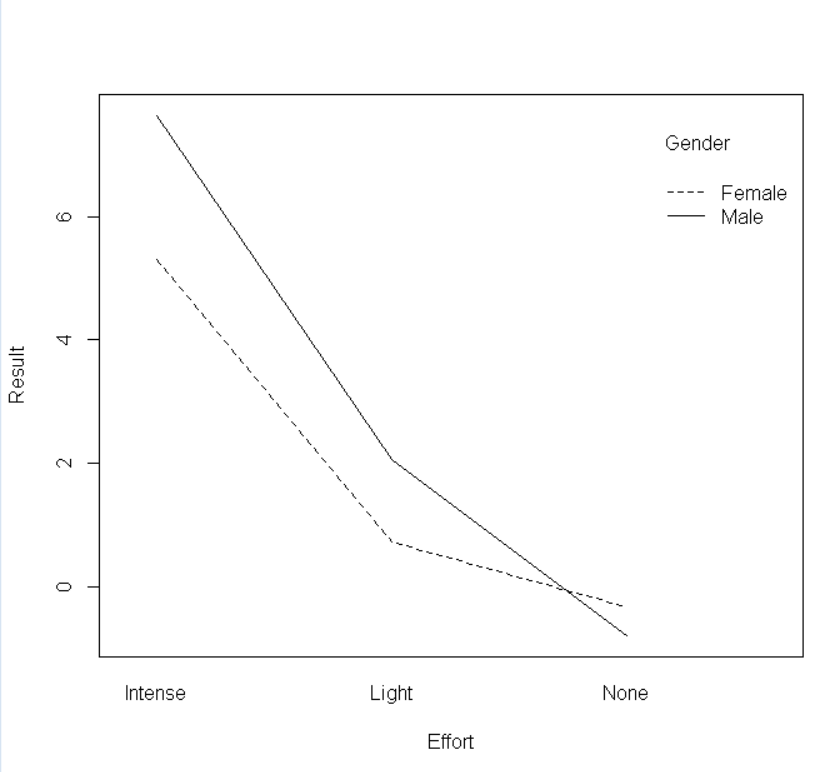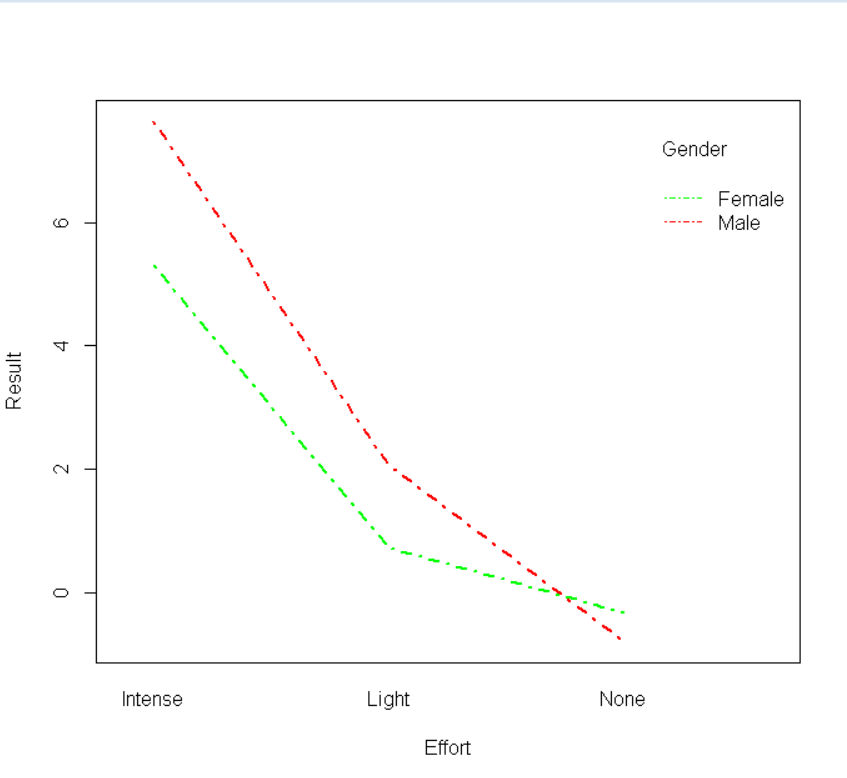# How to Create Interaction Plot in R?

• Last Updated : 26 Jan, 2022

In this article, we will discuss how to create an interaction plot in the R Programming Language.

The interaction plot shows the relationship between a continuous variable and a categorical variable in relation to another categorical variable. It lets us know whether two categorical variables have any interaction in response to a common continuous variable. If there are two parallel lines in the interaction plot, it means those two categorical variables have no interaction. Otherwise, if both lines intersect at a point that means there is an interaction between those two categorical variables.

## Create a basic Interaction Plot:

To create a basic interaction plot in the R language, we use interaction.plot() function. The interaction.plot() function helps us visualize the mean/median of the response for two-way combinations of factors. This helps us in illustrating the possible interaction. The interaction.plot() function takes x.factor, trace.factor, response, and fun as arguments and returns an interaction plot layer.

Syntax:

interaction.plot( x.factor, trace.factor, response, fun )

Parameters:

• x.factor: determines the variable whose levels will form the x-axis.
• trace.factor: determines another factor whose levels will form the traces.
• response: determines a numeric variable giving the response.
• fun: determines the statistical summary element according to which trace will be made.

### Example 1: Basic interaction plot

Here, is a basic interaction plot. The CSV file used in the example can be downloaded here.

## R

 `# import sample data to data frame ` `sample_data <- ``read.csv``(``"Sample_interaction.CSV"``) ` ` `  `# Basic Interaction Plot ` `interaction.plot``(x.factor = sample_data\$Effort, ` `                 ``trace.factor = sample_data\$gender,  ` `                 ``response = sample_data\$Result, fun = median)`

Output:### Example 2: Label Customization

To customize the x-axis and y-axis labels in the interaction plot, we use the xlab and ylab arguments of the interaction.plot() function in the R Language. To change the label of the variable in the legend of the plot, we use the trace.label argument of the interaction.plot() function in the R Language.

Syntax: interaction.plot( x.factor, trace.factor, response, fun, xlab, ylab, trace.label )

Parameters:

• xlab: determines the label for the x-axis variable.
• ylab: determines the label for the y-axis variable.
• trace.label: determines the label for the trace factor variable in legend.

Here, is a basic interaction plot with custom labels.

## R

 `# import sample data to data frame ` `sample_data <- ``read.csv``(``"Sample_interaction.CSV"``) ` ` `  `# Basic Interaction Plot with custom labels ` `interaction.plot``(x.factor = sample_data\$Effort,  ` `                 ``trace.factor = sample_data\$gender,  ` `                 ``response = sample_data\$Result,  ` `                 ``fun = median, xlab=``"Effort"``, ` `                 ``ylab=``"Result"``, trace.label=``"Gender"``)`

Output:### Example 3: Color and Shape Customization

To customize the color of the lines, we use the col parameter of the interaction.plot() function which takes a color vector as an argument. To customize the width and shape of the line, we use the lwd and lty parameters of the interaction.plot() function.

Syntax: interaction.plot( x.factor, trace.factor, response, fun, col, lwd, lty )

Parameters:

• col: determines the colors of the lines in the plot.
• lty: determines the type of line for example dashed, wedged,etc.
• lwd: determines the width of the plotline.

Here, is a basic interaction plot with custom labels, color, and shape.

## R

 `# import sample data to data frame ` `sample_data <- ``read.csv``(``"Sample_interaction.CSV"``) ` ` `  `# Basic Interaction Plot with custom labels ` `interaction.plot``(x.factor = sample_data\$Effort,  ` `                 ``trace.factor = sample_data\$gender,  ` `                 ``response = sample_data\$Result,  ` `                 ``fun = median, xlab=``"Effort"``, ` `                 ``ylab=``"Result"``, trace.label=``"Gender"``,  ` `                 ``col=``c``(``"green"``,``"red"``), ` `                 ``lty=4, lwd=2.5 )`

Output:My Personal Notes arrow_drop_up
Recommended Articles
Page :